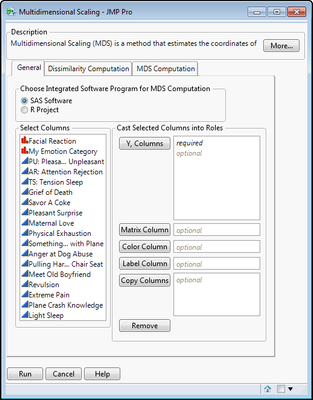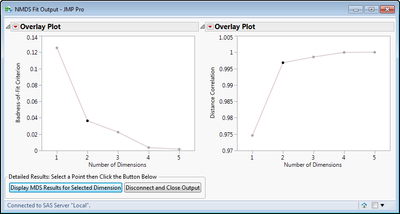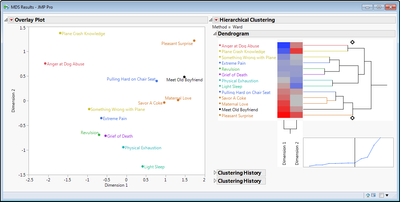Choose Language Hide Translation Bar

## Multidimensional Scaling Using R or SAS Integration

This script builds a dialog for performing Multidimensional Scaling through SAS integration or alternatively by connecting to the R project. MDS is a dimension reduction method that produces coordinates in Euclidean

space (usually 2D, 3D) that best represent the structure of a full distance/dissimilarity

matrix. MDS requires that input be a symmetric dissimilarity matrix.  Input to this application

can be data that is already in the form of a symmetric dissimilarity matrix or the dissimilarity matrix

can be computed based on the input data (where dissimilarity measures are calculated between rows of the

input data table.

##### NOTE: This script assumes that the SAS preference to use SAS variable labels for column names during import is set! Otherwise you may receive errors of variable names not found.

Refer to this blog for further information or to the ReadMe_MDSAddin.txt found in the Home Folder of the Add-In once installed.

Dialog Screen Capture:Results Screen Captures:

Summary of MDS fit at different dimensions.  Points can be selected and then results are displayed by button selection.Two-dimensional results for example Facial Expressions data showing the best fit in two-dimensions of the relationships between judging facial expressions to different stimuli/scenarios.Further details:

If the data are not already a dissimilarity matrix, one can be computed (and optionally produced).

Details can be found on these metrics in the SAS or R documentation.

PROC DISTANCE is used when integrating with SAS.

For R:

Gower is produced using the daisy() function in the R cluster package.

All others are calculated in the dist() function in the R stats package.

Once the data is in the form of a symmetric dissimilarity matrix, MDS can be computed in R using two different

R functions (details can be found in the R Documentation):

Classical MDS is computed with the cmdscale() function in the R stats package.

Kruskal's Nonmetric MDS is computed using isoMDS() function in the R MASS package.

FOr SAS, PROC MDS is used and offers more options and styles of MDS algorithms.

For R: All packages that required for this application are present in a basic R project installation

For SAS: the SAS/STAT component is required.

Several options/features are performed using JSL scripting:

- Creation of the MDS dialog for entering data and parameters.

- For R: Standardization of numeric variables if requested prior to distance matrix computation for R.

This will subtract the mean of the column and divide it by the standard deviation of the column.

- Output of the distance matrix to a JMP data table.

- Resulting dynamic plots for exploring the results of MDS.

Article Labels
Article Tags
Contributors